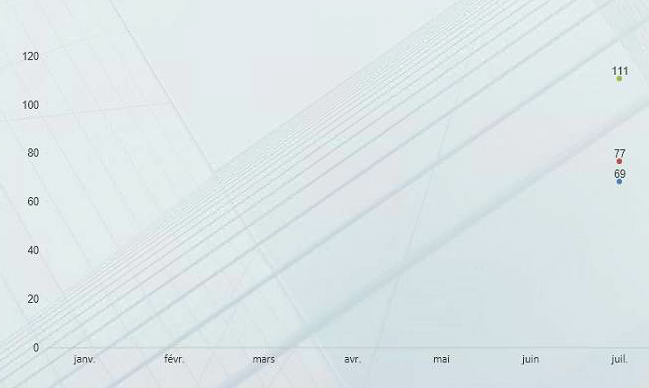# New to QlikView

Discussion board where members can get started with QlikView.

Announcements
Modernize Your QlikView Deployment webinar, Nov. 3rd. REGISTER
cancel
Showing results for
Did you mean:
HighlightedChampion

## Set analysis with networkdays problem

Hi everyone !

I'm struggling on make my expression works...

Below is my data structure:

 Date Annulation Date Création Date Début Effet Date Emission N° Quittance 04/01/2016 19/11/2015 01/01/2016 01/01/2016 2160100003 04/01/2016 04/01/2016 01/01/2015 04/01/2016 2160100192 04/01/2016 04/01/2016 01/01/2015 04/01/2016 2160100194 04/01/2016 04/01/2016 01/01/2015 04/01/2016 2160100209 04/01/2016 04/01/2016 19/01/2015 04/01/2016 2160100189 04/01/2016 04/01/2016 03/07/2015 04/01/2016 2160100210 05/01/2016 30/11/2015 01/01/2016 01/01/2016 2160100083 06/01/2016 04/01/2016 01/01/2016 04/01/2016 2160100300 06/01/2016 04/01/2016 01/01/2016 04/01/2016 2160101076 11/01/2016 04/01/2016 01/01/2016 04/01/2016 2160100263 11/01/2016 04/01/2016 01/01/2016 04/01/2016 2160100266 11/01/2016 04/01/2016 01/01/2016 04/01/2016 2160100665 11/01/2016 04/01/2016 01/01/2016 04/01/2016 2160100666

I'm calculating the avg of deadlines for issuing receipts with some conditions, with the (working) expression below:

=avg({<Equipes={'Equipe CR'},Année={\$(=Max(Année))},Mois={"<=\$(=max(Mois))"}>}aggr( if([Date Emission]>[Date Début Effet], NetWorkDays([Date Début Effet],[Date Emission])),[N° Quittance]))

I'm using a line chart and here is the result:everything is working fine until I try to filter on month field (Month field is a calendar field, my table above is linked with the calendar field with the date field "Date création"), when I select a value on month, example "July", I need the lines to show from January TO July.. but the lines are showing this:PS: THE " Année={\$(=Max(Année))},Mois={"<=\$(=max(Mois))"} "portion of my set analysis works perfectly fine on all the other charts of my app, except the one described above...

One last note: it seems that the set analysis partially works, because the chart is filtered from January to July when I select July on the field Month, but something is missing to show all the values of the line...

Youssef B.

Labels (3)

• ### Set Analysis

1 Solution

Accepted Solutions
HighlightedMVP

You might need to ignore selection in your fields inside the Aggr() function expression

``````=Avg({<Equipes = {'Equipe CR'}, Année = {\$(=Max(Année))}, Mois = {"<=\$(=max(Mois))"}>}Aggr(

If(Only({1} [Date Emission]) > Only({1} [Date Début Effet]), Only({1} NetWorkDays([Date Début Effet], [Date Emission])))

, [N° Quittance]))``````

I have ignored all selections using {1}, but you can ignore selection in certain fields based on your requirement.

8 Replies
HighlightedMVP

You might need to ignore selection in your fields inside the Aggr() function expression

``````=Avg({<Equipes = {'Equipe CR'}, Année = {\$(=Max(Année))}, Mois = {"<=\$(=max(Mois))"}>}Aggr(

If(Only({1} [Date Emission]) > Only({1} [Date Début Effet]), Only({1} NetWorkDays([Date Début Effet], [Date Emission])))

, [N° Quittance]))``````

I have ignored all selections using {1}, but you can ignore selection in certain fields based on your requirement.

HighlightedChampion

Thank you @sunny_talwar

I was trying to post the solution I found too, I replaced the if with a set analysis and used {1}:

=avg({<Equipes={'Equipe CR'},Année={\$(=Max(Année))},Mois={"<=\$(=max(Mois))"}>}aggr(sum({<[Date Création]={"=only({1}[Date Emission])>only({1}[Date Début Effet])"},Mois={"<=\$(=max(Mois))"}>} NetWorkDays([Date Début Effet],[Date Emission]))),[N° Quittance]))

HighlightedMVP

Does this expression really works? Seeing few errors in the syntax

HighlightedChampion

I was making some tests on a test app with a slightly modified expression (without Networkdays), so the one I put above I wrote it directly on the webpage, and there is only an extra bracket (the red one):

=avg({<Equipes={'Equipe CR'},Année={\$(=Max(Année))},Mois={"<=\$(=max(Mois))"}>}aggr(sum({<[Date Création]={"=only({1}[Date Emission])>only({1}[Date Début Effet])"},Mois={"<=\$(=max(Mois))"}>} NetWorkDays([Date Début Effet],[Date Emission]))),[N° Quittance]))

The real problem is when I tried this one on the real app, the numbers are completely different..

Is there something missing here too ?

HighlightedMVP

I am not sure what exactly is the expectation... my bad with not reading your expression... what I thought was an error... was not really an error.

HighlightedChampion

I was expecting to make the expression work with a set analysis instead the IF used on the first expression

That means, Replacing the Red part of your expression:

=avg({<Equipes={'Equipe CR'},Année={\$(=Max(Année))},Mois={"<=\$(=max(Mois))"}>}aggr( if(only({1}[Date Emission])>only({1}[Date Début Effet]), Only({1}NetWorkDays([Date Début Effet],[Date Emission]))),[N° Quittance]))

with the green part of this expression:

=avg({<Equipes={'Equipe CR'},Année={\$(=Max(Année))},Mois={"<=\$(=max(Mois))"}>}aggr(sum({<Date={"=only({1}[Date Emission])>only({1}[Date Début Effet])"}>} NetWorkDays([Date Début Effet],[Date Emission])),[N° Quittance]))

I thought it is working, but it is not...

Is there something missing on the expression with the green part ?

thank you

HighlightedMVP

May be try this... but you are not getting rid of the if statement... you are just moving it within your set analysis...

``=Avg({<Equipes = {'Equipe CR'}, Année = {\$(=Max(Année))}, Mois = {"<=\$(=max(Mois))"}, [N° Quittance] = {"=Only({1} [Date Emission]) > Only({1} [Date Début Effet])"}>} NetWorkDays([Date Début Effet], [Date Emission]))``

To get rid of the if statement... I would say that try to create a flag by running this in the script

``If([Date Emission]) > [Date Début Effet], 1, 0) as TrueFalseFlag``

and then this

``=Avg({<Equipes = {'Equipe CR'}, Année = {\$(=Max(Année))}, Mois = {"<=\$(=max(Mois))"}, [TrueFalseFlag] = {'1'}>} NetWorkDays([Date Début Effet], [Date Emission]))``
HighlightedChampion